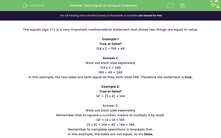# Solve Equal or Unequal Problems

In this worksheet, students will be asked to solve a range of problems that use the equal sign. Students will have to decide whether the equals sign represents a balanced mathematical statement.Key stage:  KS 2

Curriculum topic:   Number: Multiplication and Division

Difficulty level:#### Worksheet Overview

The equals sign (=) is a very important mathematical statement that shows two things are equal in value.

Example 1

True or false?

124 x 2 = 199 + 49

Work out each side separately.

124 x 2 = 248

199 + 49 = 248

In this example, the two sides are equal as they both total 248. Therefore the statement is true.

Example 2:

True or false?

14² = (5 x 8) + 144

Work out each side separately.

Remember that to square a number, means to multiply it by itself.

14² = 14 x 14 = 196

(5 x 8) + 144 = 40 + 144 = 184

Remember to complete operations in brackets first.

In this example, the sides are not equal, so it's false.

Let's have a go at spotting the false statements in the questions that follow.### What is EdPlace?

We're your National Curriculum aligned online education content provider helping each child succeed in English, maths and science from year 1 to GCSE. With an EdPlace account you’ll be able to track and measure progress, helping each child achieve their best. We build confidence and attainment by personalising each child’s learning at a level that suits them.

Get started